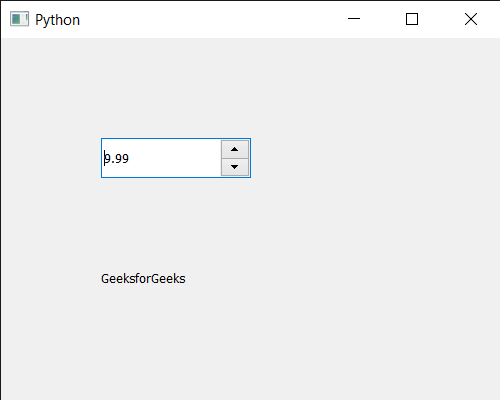# PyQt5 QDoubleSpinBox – Setting Value

• Last Updated : 14 Jul, 2020

In this article we will see how we can set value to the QDoubleSpinBox. User can set any decimal value(float) to the QDoubleSpinBox with the help of mouse and keyboard, but sometimes there is a need of setting value programmatically in order to overwrite the value entered by the user.

In order to do this we will use `setValue` method with the QDateTimeEdit object.

Attention geek! Strengthen your foundations with the Python Programming Foundation Course and learn the basics.

To begin with, your interview preparations Enhance your Data Structures concepts with the Python DS Course. And to begin with your Machine Learning Journey, join the Machine Learning - Basic Level Course

Syntax : d_spin.setValue(val)

Argument : It takes float value as argument

Return : It returns None

Below is the implementation

 `# importing libraries``from` `PyQt5.QtWidgets ``import` `*` `from` `PyQt5 ``import` `QtCore, QtGui``from` `PyQt5.QtGui ``import` `*` `from` `PyQt5.QtCore ``import` `*` `import` `sys`` ` ` ` `class` `Window(QMainWindow):`` ` `    ``def` `__init__(``self``):``        ``super``().__init__()`` ` `        ``# setting title``        ``self``.setWindowTitle(``"Python "``)`` ` `        ``# setting geometry``        ``self``.setGeometry(``100``, ``100``, ``500``, ``400``)`` ` `        ``# calling method``        ``self``.UiComponents()`` ` `        ``# showing all the widgets``        ``self``.show()`` ` `    ``# method for components``    ``def` `UiComponents(``self``):`` ` `        ``# creating double spin box``        ``d_spin ``=` `QDoubleSpinBox(``self``)`` ` `        ``# setting geometry to the double spin box``        ``d_spin.setGeometry(``100``, ``100``, ``150``, ``40``)`` ` `        ``# value``        ``value ``=` `9.99`` ` `        ``# setting current value of the double spin box``        ``d_spin.setValue(value)`` ` `        ``# creating a label``        ``label ``=` `QLabel(``"GeeksforGeeks"``, ``self``)`` ` `        ``# setting geometry to the label``        ``label.setGeometry(``100``, ``200``, ``300``, ``80``)`` ` `        ``# making label multi line``        ``label.setWordWrap(``True``)`` ` ` ` ` ` ` ` ` ` `# create pyqt5 app``App ``=` `QApplication(sys.argv)`` ` `# create the instance of our Window``window ``=` `Window()`` ` `# start the app``sys.exit(App.``exec``())`

Output :My Personal Notes arrow_drop_up